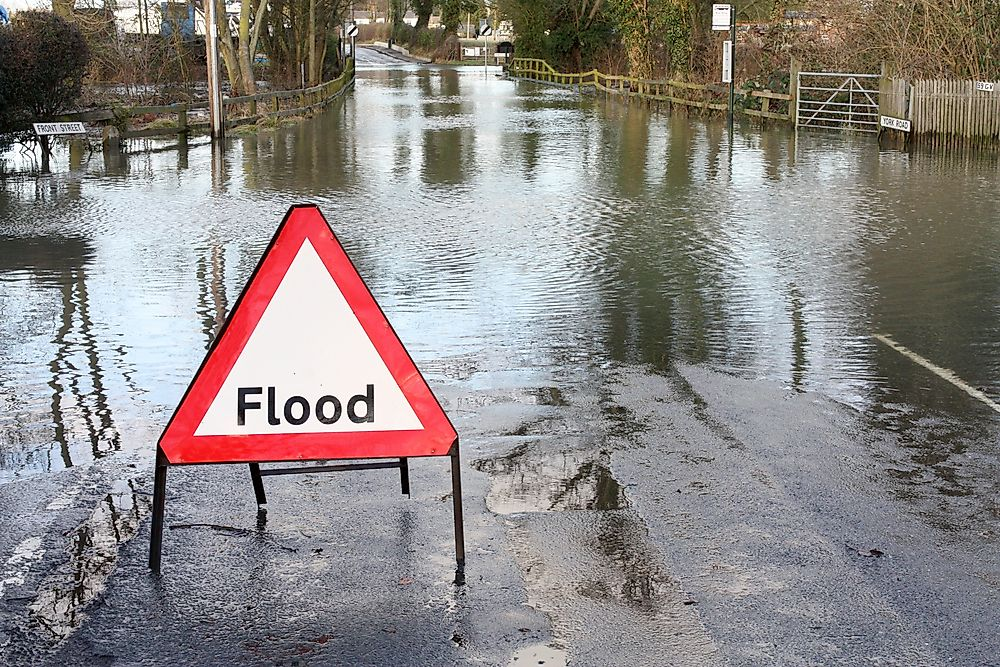# What Is A 100-Year Flood?A 100-year flood is a flooding event which has 1% likelihood of happening in any given year.

## What Is a 100-Year Flood?

Flooding is the overflow of water onto an area of land that is usually dry. Most floods occur during long periods of rainfall or are the result of rapid snow melting. Floods are not equal in terms of duration, magnitude or impact since they arise under different circumstances. A 100-year flood is a flooding event which has 1% likelihood of happening in any given year.

The United States government decided to use the 1% annual exceedance probability (AEP) flood as their basis for the National Flood Insurance Program (NFIP) in the 1960s. The 1% AEP flood seemed to be a fair balance between stringent regulations and protecting Americans against flooding. Since it has a 1 in a 100 chance of occurring in a given year, and it has a typical recurrence period of 100 years, it is known as the 100-year flood.

For rivers, the 100-year flood is expressed as a flow-rate and is based on the anticipated 100-year flow-rate. The water level can be easily plotted as an area of inundation, and the resulting map is called the 100-year floodplain. Regions near large lakes, seas, or oceans can also be flooded by a combination of waves, storm surges, and tides. The maps of coastal or riverine 100-year floodplains are essential in terms of food insurance, environmental regulations, and the issuance of building permits.

## How Is a 100-Year Flood Calculated?

Flood events are measured using a frequency technique often referred to as return period or probability of return. The return period is an estimate of the likelihood that a flood event will occur. The return period is based on historical data which denotes the average recurring interval over a given period of time. The frequency is expressed as a time interval between occurrences of a flood event and is written as a percentage which describes the tendency of a flood disaster.

Engineers and scientists use statistical probabilities to examine the occurrence of flooding. If they know that the likelihood of a flood is being exceeded or equaled, then they can assess the risk. In order to determine these probabilities, it is important to examine the annual peak streamflow measurements, which are usually measured at the streamgage. A streamgage is a place in the stream where the stream flow and the water level are measured.

## Statistical Assumptions

Scientists and engineers make numerous assumptions to determine the 100-year flood. First, they assume that all events are independent of one year another, which means that the maximum flow rate of 1985 cannot be significantly related to the observed frequency in 1986. Secondly, they assume that the flooding event should originate from the same probability distribution function. The third hypothesis dictates that the probability distribution is related to the most significant storm which occurred in the given year. They also assume that the minimum/maximum values, standard deviation, and mean are not decreasing or increasing with time, which is a notion known as stationarity.

The first hypothesis is not always valid, and it is usually tested on numerous events, while the second is assumed if the floods are observed under the same climatic conditions. The hypothesis on stationarity is hard to test, especially if the data is from one site since the distribution is always changing.

Share